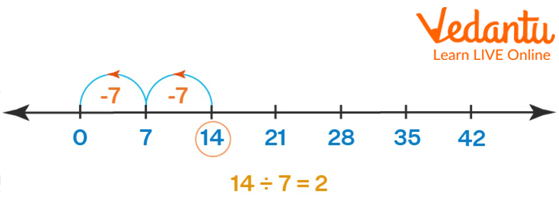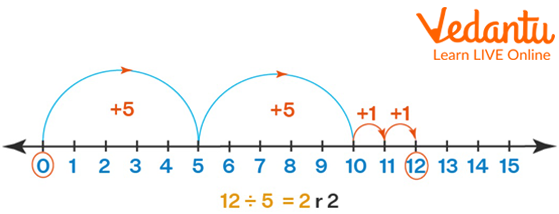Courses
Courses for Kids
Free study material
Free LIVE classes
More

# Division on Number LineLIVE
Join Vedantu’s FREE Mastercalss

## What is a Number Line?

A number line is a diagram that depicts numerals on a straight line. It is a tool for comparing and sorting numbers. It may represent any real number, including whole numbers and natural numbers. The numbers that can be arranged in the number line are the integers as well as fractions can be arranged in it.Number Line

## Number Line Representation of Division

A number line is a graphical depiction of real numbers, which includes integers, fractions, whole numbers, and so on. This is the easiest way to teach children about different operations used in maths. The division of an integer number can be done using the number line method of division. We all know that division may also be referred to as repeated subtraction. This knowledge will be used to understand the description of a division operation on a number line.

## Division Using Number Line

Consider dividing the number '14' by the number 7.' On the number line, we shall now represent 14 and 7. We will do the subtraction operation by going to the left on the number line since division is also known as repeated subtraction.

• Draw a number line with a multiple of 7 starting from 0.

• To divide 14 by 7 we will start with 14 and remove groups of 7 units at a time until we reach the number 0. Every time we subtract, we shift 7 units to the left of 14 until we reach 0.

• We can also begin with 0 as the reference point, we can proceed to the right in sets of 7 units until we reach the numeral 14.

• The quotient or the result is the number of steps of 7 units each that we went to the left of 14 to reach 0 or the number of steps of 7 units each that we moved to the right of 14 to reach 14.Division on Number Line

## Divide Using Remainders on a Number Line

In some cases when we divide the integers they leave behind a remainder as the dividend is not completely divisible by the dividend and when this happens, we obtain a non-zero remainder. Such numbers can also be represented on the number line. For example, if we divide 12 by 5 we will get a remainder of 2.Division with Remainders

## Number Line Multiplication Example

To multiply in a number line we need to form the groups of the number given and have to jump from 0 to the other number given. For example, if we are given to multiply 4 and 7 then, we have to form groups of 4 numbers and have to jump 7 times from zero the result would be 28 with groups formed as 0 to 4, 4 to 8, 8 to 12, 12 to 16, 16 to 20, 20 to 24 and 24 to 28.Multiplication on Number Line

## Conclusion

The division on the number line can be done by plotting the numbers and making the group of the divisor till we reach the dividend. It can also be done by repeated subtraction where the dividend is subtracted from the divisor till 0 or some remainder is obtained. Through the number line, we can also know the remainder we might get in the division.

## Sample Questions (Division Using Number Line Worksheet)

1. When 12 is divided by 3 then how many groups of 3 would be formed?

a. 3

b. 4

c. 5

d. 6

Ans: 4

Explanation: There would be 4 groups formed on the number line to reach 12 from 0 which would be 0 to 3, 3 to 6, 6 to 9 and 9 to 12.

2. What would be the remainder when 47 is divided by 9?

a. 2

b. 3

c. 4

d. 5

Ans: 2

Explanation: To reach 47 there would be 5 groups of 9 formed which include 0 to 9, 9 to 18, 18 to 27, 27 to 36 and 36 to 45. To reach 47 one more group of 9 is not required and only two numbers are left. So, 2 would be the remainder and 5 would be the quotient.

3. What would be the quotient when -20 is divided by 5?

a. 4

b. -4

c. none of the above

d. can be 4 or -4

Ans: -4

Explanation: To reach -20 on the number line the numbers will be plotted on the left side of the zero. So grouping of 5 numbers will be done. The groups formed will be 0 to -5, -5 to -10, -10 to -15 and -15 to -20 making a total of 4 groups on the negative side. So the quotient would be -4.

Last updated date: 28th Sep 2023
Total views: 68.1k
Views today: 2.68k

## FAQs on Division on Number Line

1. On a Number Line, how does one divide negative numbers?

Negative values can be divided on a number line by plotting the numbers towards the left-hand side of 0 when either the dividend or the divisor is negative. However, if both the dividend and the divisor are negative, the result is positive and all plottings will also be done towards the right-hand side of 0 on the number line.

2. Can addition be performed on a number line?

Yes, Addition can be performed on a number line by adding the units of the first number to the units of the second number.

3. How do we represent negative numbers on the number line?

We extend the number line towards the left-hand side of the  0 and write the negative numbers from that side.

4. What is multiplication also known as?

Multiplication is also known as repetitive addition as we need to add the number till the number of times it is mentioned in the question.

5. What is division also known as?

Division is also known as repeated subtraction as we subtract the dividend repeatedly by the divisor till we get zero as our answer. The number of times the dividend gets subtracted from the divisor is our quotient.